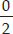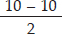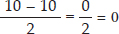Whole Numbers (Mathematics) Class 6 - NCERT Questions
Q 1.

Write the next three natural numbers after 10999.

SOLUTION:

10,999 + 1 = 11,000
11,000 + 1 = 11,001
11,001 + 1 = 11,002
∴ The next three natural numbers after 10,999 are 11,000; 11,001; 11,002.

Q 2.

Write the three whole numbers occurring just before 10001.

SOLUTION:

10,001 - 1 = 10,000
10,000 - 1 = 9,999
9,999 - 1 = 9,998
∴ The three whole numbers occurring just before 10,001 are 10,000; 9,999; 9,998

Q 3.

Which is the smallest whole number?

SOLUTION:

0 is the smallest whole number.

Q 4.

How many whole numbers are there between 32 and 53?

SOLUTION:

Since, 53 - 32 - 1 = 20
∴ There are 20 whole numbers between 32 and 53.

Q 5.

Write the successor of :
(A) 2440701
(B) 100199
(C) 1099999
(D) 2345670

SOLUTION:

(A) Successor of 2440701 is
2440701 + 1 = 2440702
(B) Successor of 100199 is
100199 + 1 = 100200
(C) Successor of 1099999 is
1099999 + 1 = 1100000
(D) Successor of 2345670 is
2345670 + 1 = 2345671

Q 6.

Write the predecessor of :
(A) 94
(B) 10000
(C) 208090
(D) 7654321

SOLUTION:

(A) The predecessor of 94 is, 94 - 1 = 93
(B) The predecessor of 10000 is, 10000 - 1 = 9999
(C) The predecessor of 208090 is,
208090 - 1 = 208089
(D) The predecessor of 7654321 is,
7654321 - 1 = 7654320

Q 7.

In each of the following pairs of numbers, state which whole number is on the left of the other number on the number line. Also write them with the appropriate sign (>, <) between them.
(A) 530, 503
(B) 370, 307
(C) 98765, 56789
(D) 9830415, 10023001

SOLUTION:

(A) 503 appears on left side of 530 on the number line. So, 530 > 503
(B) 307 appears on left side of 370 on the number line. So, 370 > 307
(C) 56789 appears on left side of 98765 on the number line. So, 98765 > 56789
(D) 9830415 appears on left side of 10023001 on the number line. So, 9830415 < 10023001

Q 8.

Which of the following statements are true (T) and which are false (F) ?
(A) Zero is the smallest natural number.
(B) 400 is the predecessor of 399.
(C) Zero is the smallest whole number.
(D) 600 is the successor of 599.
(E) All natural numbers are whole numbers.
(F) All whole numbers are natural numbers.
(G) The predecessor of a two digit number is never a single digit number.
(H) 1 is the smallest whole number.
(I) The natural number 1 has no predecessor.
(J) The whole number 1 has no predecessor.
(K) The whole number 13 lies between 11 and 12.
(L) The whole number 0 has no predecessor.
(M) The successor of a two digit number is always a two digit number.

SOLUTION:

(A) False : Since, zero is not a natural number.
(B) False : Since, 400 is the successor of 399.
(C) True
(D) True
(E) True
(F) False : All whole numbers are not natural numbers as 0 is a whole number but not a natural number.
(G) False : Since, predecessor of a two digit number i.e., 10 is a single digit number i.e., 9.
(H) False : Since, 0 is the smallest whole number.
(I) True
(J) False : Since, 0 is the predecessor of 1 in whole numbers.
(K) False : Since, 13 lies between 12 and 14.
(L) True
(M) False : Since, successor of two digit number i.e., 99 is a three digit number i.e., 100.

Q 9.

Find the sum by suitable rearrangement:
(A) 837 + 208 + 363
(B) 1962 + 453 + 1538 + 647

SOLUTION:

(A) 837 + 208 + 363 = (837 + 363) + 208
= 1200 + 208 = 1408
(B) 1962 + 453 + 1538 + 647
= (1962 + 1538) + (453 + 647)
= 3500 + 1100 = 4600

Q 10.

Find the product by suitable rearrangement:
(A) 2 × 1768 × 50
(B) 4 × 166 × 25
(C) 8 × 291 × 125
(D) 625 × 279 × 16
(E) 285 × 5 × 60
(F) 125 × 40 × 8 × 25

SOLUTION:

(A) 2 × 1768 × 50 = (2 × 50) × 1768
= 100 × 1768 = 176800
(B) 4 × 166 × 25 = (4 × 25) × 166
= 100 × 166 = 16600
(C) 8 × 291 × 125 = (8 × 125) × 291
= 1000 × 291 = 291000
(D) 625 × 279 × 16 = (625 × 16) × 279
= 10000 × 279 = 2790000
(E) 285 × 5 × 60 = 285 × (5 × 60)
= 285 × 300 = 85500
(F) 125 × 40 × 8 × 25 = (125 × 8) × (40 × 25)
= 1000 × 1000 = 1000000

Q 11.

Find the value of the following:
(A) 297 × 17 + 297 × 3
(B) 54279 × 92 + 8 × 54279
(C) 81265 × 169 - 81265 × 69
(D) 3845 × 5 × 782 + 769 × 25 × 218

SOLUTION:

(A) 297 × 17 + 297 × 3 = 297 × (17 + 3)
= 297 × 20 = 5940
(B) 54279 × 92 + 8 × 54279
= 54279 × (92 + 8) = 54279 × 100 = 5427900
(C) 81265 × 169 - 81265 × 69 = 81265 × (169 - 69)
= 81265 × 100 = 8126500
(D) 3845 × 5 × 782 + 769 × 25 × 218
= 3845 × 5 × 782 + 769 × 5 × 5 × 218
= 3845 × 5 × 782 + 3845 × 5 × 218
= 3845 × 5 × (782 + 218) = 19225 × 1000 = 19225000

Q 12.

Find the product using suitable properties.
(A) 738 × 103
(B) 854 × 102
(C) 258 × 1008
(D) 1005 × 168

SOLUTION:

By using distributive property of multiplication over addition, we get
(A) 738 × 103 = 738 × (100 + 3)
= 738 × 100 + 738 × 3 = 73800 + 2214 = 76014
(B) 854 × 102 = 854 × (100 + 2)
= 854 × 100 + 854 × 2 = 85400 + 1708 = 87108
(C) 258 × 1008 = 258 × (1000 + 8)
= 258 × 1000 + 258 × 8 = 258000 + 2064 = 260064
(D) 1005 × 168 = (1000 + 5) × 168
= 1000 × 168 + 5 × 168 = 168000 + 840 = 168840

Q 13.

A taxidriver filled his car petrol tank with 40 litres of petrol on Monday. The next day, he filled the tank with 50 litres of petrol. If the petrol costs Rs. 44 per litre, how much did he spend in all on petrol?

SOLUTION:

Petrol filled on Monday = 40 litres
Petrol filled on next day = 50 litres
Total petrol filled = (40 + 50) litres = 90 litres
Now, cost of 1 litre of petrol = ₹ 44
Cost of 90 litres of petrol = ₹ (44 × 90) = ₹ 3960
Therefore, the taxidriver spent ₹3960 on petrol.

Q 14.

A vendor supplies 32 litres of milk to a hotel in the morning and 68 litres of milk in the evening. If the milk costs ₹ 15 per litre, how much money is due to the vendor per day?

SOLUTION:

Supply of milk in the morning = 32 litres
Supply of milk in the evening = 68 litres
Total supply of milk = (32 + 68) litres = 100 litres
Now, cost of 1 litre of milk =₹ 15
Cost of 100 litres of milk = ₹ (15 × 100) = ₹ 1500
Therefore, ₹ 1500 is due to the vendor per day.

Q 15.

Match the following:
(I) 425 × 136 = 425 × (6 + 30 +100)      (A) Commutativity under multiplication.
(ii) 2 × 49 × 50 = 2 × 50 × 49      (B) Commutativity under addition.
(iii) 80 + 2005 + 20 = 80 + 20 + 2005      (C) Distributivity of multiplication over addition.

SOLUTION:

(I) → (C); (ii) → (A); (iii) → (B)
(I) 425 × 136 = 425 × (6 + 30 + 100)
This is the principle of distributivity of multiplication over addition.
(ii) 2 × 49 × 50 = 2 × 50 × 49
This is the principle of commutativity under multiplication.
(iii) 80 + 2005 + 20 = 80 + 20 + 2005
This is the principle of commutativity under addition.

Q 16.

Which of the following will not represent zero?
(A) 1 + 0
(B) 0 × 0
(C)(D)SOLUTION:

(A) 1 + 0 = 1
(B)0 × 0 = 0
(C)(D)Thus, (A) does not represent zero.

Q 17.

If the product of two whole numbers is zero, can we say that one or both of them will be zero? Justify through examples.

SOLUTION:

Yes, if we multiply any number with zero, then the product will be zero.
i.e., 2 × 0 = 0, 5 × 0 = 0, 9 × 0 = 0
If both numbers are zero, then the product will also be zero. i.e., 0 × 0 = 0

Q 18.

If the product of two whole numbers is 1, can we say that one or both of them will be 1? Justify through examples.

SOLUTION:

No, if only one number be 1, then the product cannot be 1.
i.e., 5 × 1 = 5, 4 × 1 = 4, 8 × 1 = 8
If both numbers are 1, then the product is 1 i.e., 1 × 1 = 1

Q 19.

Find using distributive property :
(A) 728 × 101
(B) 5437 × 1001
(C) 824 × 25
(D) 4275 × 125
(E) 504 × 35

SOLUTION:

(A) 728 × 101 = 728 × (100 + 1)
= 728 × 100 + 728 × 1 = 72800 + 728 = 73528
(B) 5437 × 1001 = 5437 × (1000 + 1)
= 5437 × 1000 + 5437 × 1
= 5437000 + 5437 = 5442437
(C) 824 × 25 = 824 × (20 + 5) = 824 × 20 + 824 × 5
= 16480 + 4120 = 20600
(D) 4275 × 125 = 4275 × (100 + 20 + 5)
= 4275 × 100 + 4275 × 20 + 4275 × 5
= 427500 + 85500 + 21375 = 534375
(E) 504 × 35 = (500 + 4) × 35 = 500 × 35 + 4 × 35
= 17500 + 140 = 17640

Q 20.

Study the pattern :
1 × 8 + 1 = 9 1234 × 8 + 4 = 9876
12 × 8 + 2 = 98 12345 × 8 + 5 = 98765
123 × 8 + 3 = 987
Write the next two steps. Can you say how the pattern works?
(Hint: 12345 = 11111 + 1111 + 111 + 11 + 1).

SOLUTION:

The next two steps are :
123456 × 8 + 6 = 987654
1234567 × 8 + 7 = 9876543
Pattern works like this :
1 × 8 + 1 = 9
12 × 8 + 2 = 98
123 × 8 + 3 = 987
1234 × 8 + 4 = 9876
12345 × 8 + 5 = 98765
123456 × 8 + 6 = 987654
1234567 × 8 + 7 = 9876543